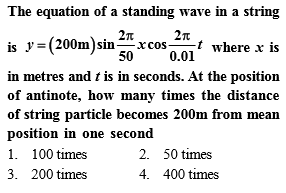# NEET Physics Waves Questions Solved

equation of wave is given by

y = 0.4 sin(314t - 3.14x), where x and y are in meter and t is in second. The speed of the wave is

1.  50 m/s

2.  100 m/s

3.  314 m/s

4.  3.14 m/s

Complete Question Bank + Test Series
Complete Question Bank

Difficulty Level:

A plane progressive wave cannot be represented by

1.

2.

3.

4.

Complete Question Bank + Test Series
Complete Question Bank

Difficulty Level:

The [phase difference between the prongs of a tuning fork is

1.

2.

3.

4.  $5\mathrm{\pi }$

Complete Question Bank + Test Series
Complete Question Bank

Difficulty Level:

1.

2.

3.

4.  $\sqrt{{\mathrm{E}}_{\mathrm{a}}}$

Complete Question Bank + Test Series
Complete Question Bank

Difficulty Level:

When a wave is reflected from a denser medium the change in phase is

1.  0

2.

3.

4.  $3\mathrm{\pi }$

Complete Question Bank + Test Series
Complete Question Bank

Difficulty Level:

In a stationary wave along a string, the strain is

1.  zero at the antinodes

2.  maximum at the antinodes

3.  zero at the nodes

4.  maximum at the nodes

Complete Question Bank + Test Series
Complete Question Bank

Difficulty Level:

A source of sound moves away with velocitv of sound from a stationary observer. Then the frequency of the sound heard by the observer

1. remains the same

2. is doubled

3. is halved

4. becomes infinity

Complete Question Bank + Test Series
Complete Question Bank

Difficulty Level:

the longitudinal waves can travel

1.  only in liquids

2.  only in solids

3.  only in gases

4.  In liquids, solids, and gases

Complete Question Bank + Test Series
Complete Question Bank

Difficulty Level:

1.  5m

2.  20m

3.  15

4.  30m